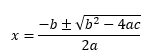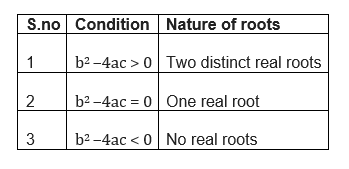Table of Content

$ax^2 +bx+c=0$,
roots are given by$x=\frac{-b+\sqrt{b^{2}-4ac}}{2a}$
and
$x=\frac{-b-\sqrt{b^{2}-4ac}}{2a}$
This has been derived using the square method as below
$ax^2 +bx+c=0$
$x^2 +\frac {b}{a} x+\frac {c}{a}=0$
$(x+ \frac {b}{2a})^2 - (\frac {b}{2a})^2 + \frac {c}{a}=0$
$(x+ \frac {b}{2a})^2=\frac {(b^2-4ac)}{4a^2}$
$(x+ \frac {b}{2a})= \pm \frac {\sqrt {b^2-4ac}}{2a}$
$x=\frac{-b \pm \sqrt{b^{2}-4ac}}{2a}$
For $b^2 -4ac > 0$, Quadratic equation has two real roots of different value
For $b^2 -4ac =0$, quadratic equation has one real root
For $b^2 -4ac < 0$, no real roots for quadratic equation
Example
$3x^2 - 5x + 2 = 0$
Solution step 1 Here a =3 ,b=-5 and c=2
step 2 Substituting in these formula
$x=\frac{-b+\sqrt{b^{2}-4ac}}{2a}$
and
$x=\frac{-b-\sqrt{b^{2}-4ac}}{2a}$
step 3 we get the root as 2/3 and 1

## Nature of roots of Quadratic equation

$b^2 -4ac$ is called the discriminant of the quadratic equation## Problem based on discriminant of a quadratic equation

Example
The sum of the ages of two friends A and B is 20 years. Four years ago, the product of their ages in years was 48.
Solution
Here we need to advise if that is a possible condition

Let the age of A be x years.
then the age of the B will be (20 - x) years.
Now 4 years ago,
Age of A = (x - 4) years
Age of N = (20 - x - 4) = (16 - x) years
So we get that,
$(x - 4) (16 - x) = 48$
$16x - x^2 - 64 + 4x = 48$
$x^2 - 20x + 112 = 0$
Comparing this equation with $ax^2 + bx + c = 0$, we get
a = 1, b = -20 and c = 112
Discriminant = $b^2 - 4ac$
= (-20)2 - 4 * 112
= 400 - 448 = -48

$b^2 - 4ac <0$ Therefore, there will be no real solution possible for the equations. Such type of condition doesn't exist

Example
If in a quadratic equation the coefficient of x is zero, then the quadratic equation has no real roots
Solution
False, since the discriminant in this case is -4ac which can still be non-negative
if a and c are of opposite signs or if one of a or c is zero.

## Solved examples

Example-1
Find the roots of the quadratic equation $x^2 -6x=0$
Solution
There is no constant term in this quadratic equation, we can x as common factor
$x(x-6)=0$
So roots are x=0 and x=6

Example-2
Solve the quadratic equation $x^2 -16=0$
Solution
$x^2 -16=0$
$x^2 =16$
or$x=4 \; or \; -4$

Example-3
Solve the quadratic equation by factorization method $x^2 -x -20=0$
Solution
Step 1 First we need to multiple the coefficient a and c.In this case =1X-20=-20. The possible multiple are 4,5 ,2,10
Step 2 The multiple 4,5 suite the equation
$x^2-5x+4x-20=0$
$x(x-5)+4(x-5)=0$
$(x+4)(x-5)=0$
or $x=-4 \; or \; 5$

Example-4
Solve the quadratic equation by Quadratic method $x^2 -3x-18=0$
Solution
$ax^2 +bx+c=0$,
roots are given by
$x=\frac{-b+\sqrt{b^{2}-4ac}}{2a}$
and
$x=\frac{-b-\sqrt{b^{2}-4ac}}{2a}$
Here a=1
b=-3
c=-18
Substituting these values,we get
$x=6 \; and \; -3$

• $x^{2} - 5 x - 14 = 0$
• $x^{2} - 3 x - 54 = 0$
• $x^{2} - 14 x + 45 = 0$
• $x^{2} - 8 x - 25 = -5$
• $x^{2} - 11 x + 18 = 0$
• $x^{2} - 13 x + 42 = 0$
• $x^{2} + 3 x - 54 = 0$
• $x^{2} + 2 x +2 = 50$
• $x^{2} - 16 x + 63 = 0$
• $x^{2} - 15 x + 56 = 0$
• $x^{2} + 7 x + 18 = 48$

### Quiz Time

Question 1
What is the Solution of Quadratic equation
$x^2 + 16=0$
(A) 4,-4
(B) No real roots
(C) 4
(D) -4
Question 2
What is the solution of Quadratic equation
$2x^2 - 32=0$
(A) 4,0
(B) -4,0
(C) 4,-4
(D) No real roots
Question 3
What is the solution of Quadratic equation
$6x^2 - 7x + 2 = 0$
(A) 2/3,1/2
(B) 1/3,1/2
(C) -1/2,1/3
(D) -2/3,1/2
Question 4
which of following statement is True
(A) Every quadratic equation has at least two roots
(B) Every quadratic equations has at most two roots.
(C) Every quadratic equation has exactly one root
(D) none of these
Question 5
Which of the following equations has 2 as a root?
(A) $y^2 - 4y + 5 = 0$
(B) $y^2 + 3y - 12 = 0$
(C)$3y^2 - 6y - 2 = 0$
(D) $2y^2 - 7y + 6 = 0$
Question 6
Is .3 root of quadratic equation?
$x^2 - .9=0$
(A) True
(B) FalseGo back to Class 10 Main Page using below links

### Practice Question

Question 1 What is $1 - \sqrt {3}$ ?
A) Non terminating repeating
B) Non terminating non repeating
C) Terminating
D) None of the above
Question 2 The volume of the largest right circular cone that can be cut out from a cube of edge 4.2 cm is?
A) 19.4 cm3
B) 12 cm3
C) 78.6 cm3
D) 58.2 cm3
Question 3 The sum of the first three terms of an AP is 33. If the product of the first and the third term exceeds the second term by 29, the AP is ?
A) 2 ,21,11
B) 1,10,19
C) -1 ,8,17
D) 2 ,11,20